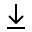MathBin Point Member

A point is a zero-dimensional object - it has zero size (zero length, zero width, and zero height). A point represents location (or position) in Euclidean space. It is displayed as a dot and named by an italic capital letter. Examples of points are:

• P = 5 for one dimension
• P = (2,3) in two dimensions
• P = (3,4,5) for three dimensions
Bins in Two Dimensions